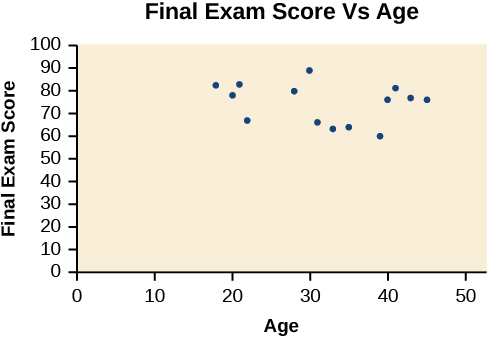# 4.3 Fitting linear models to data

 Page 1 / 14
In this section you will:
• Draw and interpret scatter diagrams.
• Use a graphing utility to find the line of best fit.
• Distinguish between linear and nonlinear relations.
• Fit a regression line to a set of data and use the linear model to make predictions.

A professor is attempting to identify trends among final exam scores. His class has a mixture of students, so he wonders if there is any relationship between age and final exam scores. One way for him to analyze the scores is by creating a diagram that relates the age of each student to the exam score received. In this section, we will examine one such diagram known as a scatter plot.

## Drawing and interpreting scatter plots

A scatter plot is a graph of plotted points that may show a relationship between two sets of data. If the relationship is from a linear model , or a model that is nearly linear, the professor can draw conclusions using his knowledge of linear functions. [link] shows a sample scatter plot.A scatter plot of age and final exam score variables

Notice this scatter plot does not indicate a linear relationship . The points do not appear to follow a trend. In other words, there does not appear to be a relationship between the age of the student and the score on the final exam.

## Using a scatter plot to investigate cricket chirps

[link] shows the number of cricket chirps in 15 seconds, for several different air temperatures, in degrees Fahrenheit Selected data from http://classic.globe.gov/fsl/scientistsblog/2007/10/. Retrieved Aug 3, 2010 . Plot this data, and determine whether the data appears to be linearly related.

 Chirps 44 35 20.4 33 31 35 18.5 37 26 Temperature 80.5 70.5 57 66 68 72 52 73.5 53

Plotting this data, as depicted in [link] suggests that there may be a trend. We can see from the trend in the data that the number of chirps increases as the temperature increases. The trend appears to be roughly linear, though certainly not perfectly so.

## Finding the line of best fit

Once we recognize a need for a linear function to model that data, the natural follow-up question is “what is that linear function?” One way to approximate our linear function is to sketch the line that seems to best fit the data. Then we can extend the line until we can verify the y -intercept. We can approximate the slope of the line by extending it until we can estimate the $\text{\hspace{0.17em}}\frac{\text{rise}}{\text{run}}.$

## Finding a line of best fit

Find a linear function that fits the data in [link] by “eyeballing” a line that seems to fit.

On a graph, we could try sketching a line. Using the starting and ending points of our hand drawn line, points (0, 30) and (50, 90), this graph has a slope of

$\begin{array}{l}\hfill \\ \begin{array}{l}m=\frac{60}{50}\hfill \\ \text{\hspace{0.17em}}\text{\hspace{0.17em}}\text{\hspace{0.17em}}\text{\hspace{0.17em}}=1.2\hfill \end{array}\hfill \end{array}$

and a y -intercept at 30. This gives an equation of

$T\left(c\right)=1.2c+30$

where $\text{\hspace{0.17em}}c\text{\hspace{0.17em}}$ is the number of chirps in 15 seconds, and $\text{\hspace{0.17em}}T\left(c\right)\text{\hspace{0.17em}}$ is the temperature in degrees Fahrenheit. The resulting equation is represented in [link] .

## Recognizing interpolation or extrapolation

While the data for most examples does not fall perfectly on the line, the equation is our best guess as to how the relationship will behave outside of the values for which we have data. We use a process known as interpolation when we predict a value inside the domain and range of the data. The process of extrapolation is used when we predict a value outside the domain and range of the data.

#### Questions & Answers

A laser rangefinder is locked on a comet approaching Earth. The distance g(x), in kilometers, of the comet after x days, for x in the interval 0 to 30 days, is given by g(x)=250,000csc(π30x). Graph g(x) on the interval [0, 35]. Evaluate g(5)  and interpret the information. What is the minimum distance between the comet and Earth? When does this occur? To which constant in the equation does this correspond? Find and discuss the meaning of any vertical asymptotes.
Kaitlyn Reply
The sequence is {1,-1,1-1.....} has
amit Reply
circular region of radious
Kainat Reply
how can we solve this problem
Joel Reply
Sin(A+B) = sinBcosA+cosBsinA
Eseka Reply
Prove it
Eseka
Please prove it
Eseka
hi
Joel
June needs 45 gallons of punch. 2 different coolers. Bigger cooler is 5 times as large as smaller cooler. How many gallons in each cooler?
Arleathia Reply
7.5 and 37.5
Nando
find the sum of 28th term of the AP 3+10+17+---------
Prince Reply
I think you should say "28 terms" instead of "28th term"
Vedant
the 28th term is 175
Nando
192
Kenneth
if sequence sn is a such that sn>0 for all n and lim sn=0than prove that lim (s1 s2............ sn) ke hole power n =n
SANDESH Reply
write down the polynomial function with root 1/3,2,-3 with solution
Gift Reply
if A and B are subspaces of V prove that (A+B)/B=A/(A-B)
Pream Reply
write down the value of each of the following in surd form a)cos(-65°) b)sin(-180°)c)tan(225°)d)tan(135°)
Oroke Reply
Prove that (sinA/1-cosA - 1-cosA/sinA) (cosA/1-sinA - 1-sinA/cosA) = 4
kiruba Reply
what is the answer to dividing negative index
Morosi Reply
In a triangle ABC prove that. (b+c)cosA+(c+a)cosB+(a+b)cisC=a+b+c.
Shivam Reply
give me the waec 2019 questions
Aaron Reply

### Read also:

#### Get the best Algebra and trigonometry course in your pocket!

Source:  OpenStax, Algebra and trigonometry. OpenStax CNX. Nov 14, 2016 Download for free at https://legacy.cnx.org/content/col11758/1.6
Google Play and the Google Play logo are trademarks of Google Inc.

Notification Switch

Would you like to follow the 'Algebra and trigonometry' conversation and receive update notifications?ByByBy